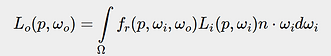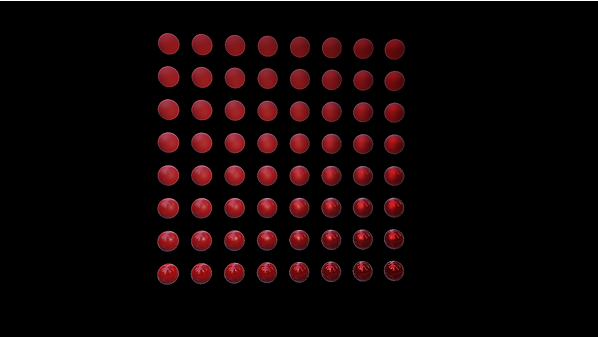top of page##### Physics-Based Rendering Model

I have implemented PBR shading model based on the tutorial posted on the LEARN OpenGL as well as the original paper that Disney published.  There are detailed theory and code there, so I'll just briefly go over what I did.

For realistic rendering, we have to simulate the reflectance equation as accurate as possible. The reflectance equation is shown below.The integral indicates the sum of all the direction the lights come from. Function Li() is the incoming right source. Function fr() is the BRDF that decides how the incoming right will get processed.

For this PBR, I have adopted Cook-Torrance BRDF which is shown below.The Cook-Torrance model can be also separated into 3 functions. D for normal distribution function, G for geometry function F for fresnel equation. I'll omit the detailed information because there is a detailed explanation in the original tutorial. By using those models, I can modify two 0~1 variables roughness and metallicness. Below are the PBR shading with different roughness and metallicness.Roughness

Metallicness

##### Image Base Rendering

The PBR I implemented is only considered direct lights. In the real world, lights coming from not just the directions but from many different directions because lights can be bouncing around and the reflected lights can be also considered as light sources. In order to calculate those indirect lights, we have to consider many more points in the space, and this is not realistic processing on the fly. Instead of that, the model I used simplified the equation, and precompute those equations and stored as texturesDiffuse Part

Specular Part

First, we separate the reflectance equation for indirect light to the diffuse part and specular part. Then we calculate and store the diffuse part as diffuse irradiance texture based on the environment cube map.Environment Cube MapFor the specular part, things get a little bit complicated. Because it is impossible to convolute the map for the specular part as a texture due to the fact it requires two different angles which means we have to prepare n^2 textures. We use split sum approximation to simplify the process. By doing so, we only have to prepare several secular maps with different LOD and BRDF lookup texture.Environment Cube MapSpecular Cube MapsBRDF Lookup Texture

Know we have precomputed all the texture that is required for IBL. If we add those textures to the PBR, it will look like this.If I take the PBR texture from FreePBR, and applied to the scene, it will look like this.The webpages and pictures I took as references are below.

bottom of page